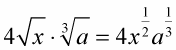##### Linear Algebra For Dummies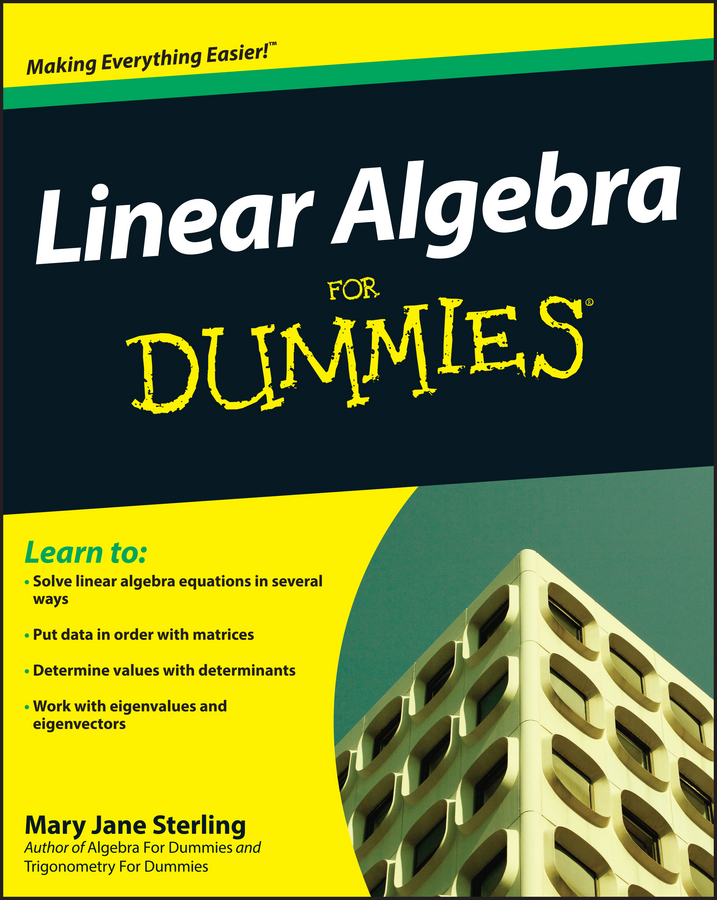## Combining square roots

Square roots, which use the radical symbol, are nonbinary operations — operations which involve just one number — that ask you, “What number times itself gives you this number under the radical?” Finding square roots is a relatively common operation in algebra, but working with and combining the roots isn’t always so clear.

Expressions with radicals can be multiplied or divided as long as the root power or value under the radical is the same. Expressions with radicals cannot be added or subtracted unless both the root power and the value under the radical are the same.

When you find square roots, the symbol for that operation is called a radical. The root power refers to the number outside and to the upper left of the radical. If there is no number, you assume that the root power is 2.

When it comes to combining radicals, there are just a couple of simple rules to remember:
• Addition and subtraction can be performed if the root power and the value under the radical are the same.

Examples:

These radicals cannot be combined because the operation is addition, and the value under the radical is not the same: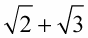These radicals can be combined because the root power and the numbers under the radical are the same:These radicals cannot be combined because the operation is subtraction, and the root power isn’t the same: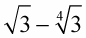• Multiplication and division can be performed if the root powers are the same.

Examples:

These radicals can be combined because the operation is multiplication, and the root power is the same: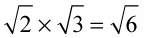These radicals can be combined because the operation is division, and the root power is the same: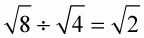These radicals cannot be combined because the operation is division, and the root power isn’t the same: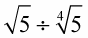## Converting square roots to exponents

Finding square roots and converting them to exponents is a relatively common operation in algebra. Square roots, which use the radical symbol, are nonbinary operations — operations which involve just one number — that ask you, “What number times itself gives you this number under the radical?” To convert the square root to an exponent, you use a fraction in the power to indicate that this stands for a root or a radical.

When you find square roots, the symbol for that operation is a radical, which looks like this: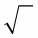When changing from radical form to fractional exponents, remember these basic forms:

• The nth root of a can be written as a fractional exponent with a raised to the reciprocal of that power.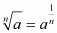• When the nth root of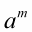is taken, it’s raised to the 1/n power. When a power is raised to another power, you multiply the powers together, and so the m (otherwise written as m/1) and the 1/n are multiplied together.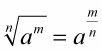Use fractions in the powers to indicate that the expression stands for a root or a radical.

Here are some examples of changing radical forms to fractional exponents: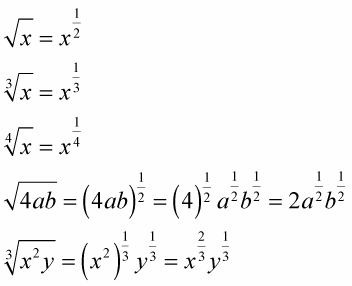When raising a power to a power, you multiply the exponents, but the bases have to be the same.

Because raising a power to a power means that you multiply exponents (as long as the bases are the same), you can simplify the following expressions: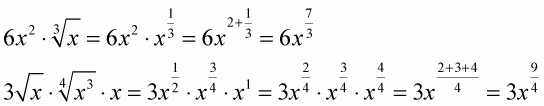Leave the exponent as 9/4. Don’t write it as a mixed number.

The following example can’t be combined because the bases are not the same: